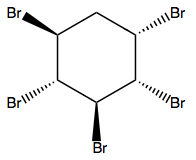# Problem: Compound C has an [a] D = +28°.a) Draw the structure of compund D, which is isomer of C and features an [a]  D = -28°.b) Calculate the [a] D of  a 25/75 mixture of derivatives C and D, respectively.c) Draw the structure of a compound E, which is an isomer of C and D, and features an [a]  D = 0°

###### Problem Details

Compound C has an [a] = +28°.

a) Draw the structure of compund D, which is isomer of C and features an [a]  D = -28°.

b) Calculate the [a] D of  a 25/75 mixture of derivatives C and D, respectively.

c) Draw the structure of a compound E, which is an isomer of C and D, and features an [a]  D = 0°